Test: Coordination Chemistry- 3

# Test: Coordination Chemistry- 3

Test Description

## 30 Questions MCQ Test Inorganic Chemistry | Test: Coordination Chemistry- 3

Test: Coordination Chemistry- 3 for Chemistry 2022 is part of Inorganic Chemistry preparation. The Test: Coordination Chemistry- 3 questions and answers have been prepared according to the Chemistry exam syllabus.The Test: Coordination Chemistry- 3 MCQs are made for Chemistry 2022 Exam. Find important definitions, questions, notes, meanings, examples, exercises, MCQs and online tests for Test: Coordination Chemistry- 3 below.
Solutions of Test: Coordination Chemistry- 3 questions in English are available as part of our Inorganic Chemistry for Chemistry & Test: Coordination Chemistry- 3 solutions in Hindi for Inorganic Chemistry course. Download more important topics, notes, lectures and mock test series for Chemistry Exam by signing up for free. Attempt Test: Coordination Chemistry- 3 | 30 questions in 90 minutes | Mock test for Chemistry preparation | Free important questions MCQ to study Inorganic Chemistry for Chemistry Exam | Download free PDF with solutions
 1 Crore+ students have signed up on EduRev. Have you?
Test: Coordination Chemistry- 3 - Question 1

### The correct order of wavelength (λmax) of the halide to metal charge-transfer band of [Co(NH3)5Cl]2+ (I), [Co(NH3)5Br]2+ (II) and [Co(NH3)5I]2+ (III), is

Detailed Solution for Test: Coordination Chemistry- 3 - Question 1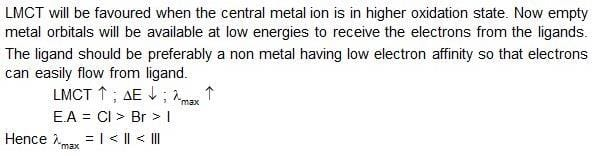Test: Coordination Chemistry- 3 - Question 2

### The purple colour of iodine vapours is due to:

Detailed Solution for Test: Coordination Chemistry- 3 - Question 2

To Know the color of the halogens you have to know the MO diagram of X2 (X=F,Cl,Br,I).

The color is due to the transition from π* to σ* in the MO diagram of I2.

The electrons of π* absorb suitable frequency of light and go to sigma* molecular orbital.

Transition from HOMO to LUMO and the complimentary color is observed.

I2 has Violet color ,it is the complimentary color of orange-yellow region,so,the light of orange yellow region is absorbed and violet color is seen.

Test: Coordination Chemistry- 3 - Question 3

### The ground state term symbols for high spin d5s1 and d5 configurations, respectively are:

Test: Coordination Chemistry- 3 - Question 4

The volume (in mL) of 0.1 M AgNO3 required for complex precipitation of chloride ions present in 30 mL of 0.01 M solution of [Cr(H2O)5Cl]Cl2, as silver chloride is close to

Detailed Solution for Test: Coordination Chemistry- 3 - Question 4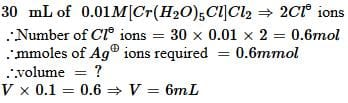Test: Coordination Chemistry- 3 - Question 5

The strongest ligand in spectro–chemical series is:

Test: Coordination Chemistry- 3 - Question 6

[NiCl2(PPh3)2] is paramagnetic with μeff = 2.9 BM. All the four ligands are monodentate. The geometry of the molecule is:

Test: Coordination Chemistry- 3 - Question 7

A solution containing 2.675 g of CoCl3 · 6NH3 (molar mass = 267.5 g mol–1) is passed through a cation exchanger. The chloride ions obtained in solution were treated with excess of AgNO3 to give 4.78 g of AgCl (molar mass = 143.5 g mol–1). The formula of the complex is
(At. Mass of Ag = 108 u)

Test: Coordination Chemistry- 3 - Question 8

Ground term symbol for Mn2+ is:

Test: Coordination Chemistry- 3 - Question 9

For which of the following ions is the colour in aqueous solution not caused by any d–d transition?

Test: Coordination Chemistry- 3 - Question 10

The cyano complex that exhibit highest value of paramagnetism is:

Test: Coordination Chemistry- 3 - Question 11

The ionisation isomer of [Cr(H2O)4Cl(NO2)C] is

Detailed Solution for Test: Coordination Chemistry- 3 - Question 11

Ionization isomers are identical except for a ligand has exchanged places with anion or neutral molecule that was originally outside the coordination complex.

Thus, in Ionization isomerism the central ion and the other ligands are identical.

So, according to the definition, Cl- is replaced by NO2-​ in ionization sphere.

Thus the correct answer is option B.

Test: Coordination Chemistry- 3 - Question 12

An excess of AgNO3 is added to 100 mL of a 0.01 M solution of dichlorotetraaquachromium(III) chloride. The number of moles of AgCl precipitated would be

Test: Coordination Chemistry- 3 - Question 13

Synergic bonding is absent in:

Test: Coordination Chemistry- 3 - Question 14

The pair of compounds having metals in their highest oxidation state is

Test: Coordination Chemistry- 3 - Question 15

Which of the following complex is high spin?

Test: Coordination Chemistry- 3 - Question 16

The absorption of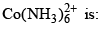Test: Coordination Chemistry- 3 - Question 17

Correct relat ionship between pairing energy (P) and C.F.S.E (∆0) in complex ion [Ir(H2O)6]3+ is:

Test: Coordination Chemistry- 3 - Question 18

In which of the following pairs, both the complexes have the same hybridization?

Test: Coordination Chemistry- 3 - Question 19

The value of CFSE (∆0) for complexes given below follow the order:

(I) [Co(NH3)6]3+
(II) [Rh(NH3)6]3+
(III) [Ir(NH3)6]3+

Test: Coordination Chemistry- 3 - Question 20

Cr3+ form for complexes with four different ligands which are [Cr(Cl)6]3–,[Cr(H2O)6]3+, [Cr(NH3)6]3+ and [Cr(CN)6]3–. The order of CFSE (∆0) in these complexes is in the order:

Test: Coordination Chemistry- 3 - Question 21

The d–orbital, which are stabilized in an octahedral magnetic field, are:

Test: Coordination Chemistry- 3 - Question 22

Total number of geometrical isomers for the complex [RhCl(CO)(PPh3)(NH3)]is

Test: Coordination Chemistry- 3 - Question 23

Which of the following is correct arrangement of ligand in terms of the Dq values of their complexes with any particular ‘hard’ metal ion:

Test: Coordination Chemistry- 3 - Question 24

The extent of crystal field splitting in octahedral complexes of the given metal with particular weak field ligand are:

Test: Coordination Chemistry- 3 - Question 25

The molar absorptivity at λmax is minimum for:

Test: Coordination Chemistry- 3 - Question 26

A magnetic moment of 1.73 BM will be shown by one among the following

*Multiple options can be correct
Test: Coordination Chemistry- 3 - Question 27

Consider the following two reactions: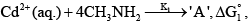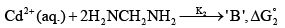According to given information the correct statement (s) is/are:

Test: Coordination Chemistry- 3 - Question 28

The value of n for the complex [Fe(CO)4(SiMe3)]n satisfying the 18-electron rule is

Detailed Solution for Test: Coordination Chemistry- 3 - Question 28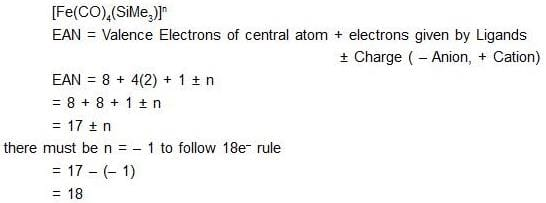*Multiple options can be correct
Test: Coordination Chemistry- 3 - Question 29

Select correct statement (s) regarding octahedron complex having CFSE = –1.2∆0.

Test: Coordination Chemistry- 3 - Question 30

Which has maximum paramagnetic character?

## Inorganic Chemistry

36 videos|71 docs|16 tests
 Use Code STAYHOME200 and get INR 200 additional OFF Use Coupon Code
Information about Test: Coordination Chemistry- 3 Page
In this test you can find the Exam questions for Test: Coordination Chemistry- 3 solved & explained in the simplest way possible. Besides giving Questions and answers for Test: Coordination Chemistry- 3, EduRev gives you an ample number of Online tests for practice

## Inorganic Chemistry

36 videos|71 docs|16 tests